##### Suplementary notes on select topics in physics and electronics
 Standing waves in a transmission line New Operating modes of 8255A PPI New Select theory of pn junction diode Resonance in series LCR circuit Wave packet and its dynamics Theory of frequency modulation
###### Basic Diode Theory

When a single semiconductor crystal is doped with trivalent impurity atoms on one side and with pentavalent impurity atoms on the other side, a p-n junction is formed between the resultant p and n regions. A semiconductor device which utilizes the unique properties that result from such a junction is called a p-n diode.
In an unbiased p-n junction diode (not connected to an external voltage source), a so called depletion region exists on both sides of the junction, which is depleted or devoid of any charge carriers (free electrons and holes). A potential energy barrier exists across this region which prevents the flow of carriers across the junction after equilibrium has been reached. Thus the diode does not conduct.
When the diode is forward biased (the p side being connected to the +ve terminal of an external battery and the n side to the -ve terminal of the battery), the internal barrier field decreases (with a corresponding decrease in the width of the depletion region), as a result of which the majority charge carriers have sufficient energy to cross the barrier and constitute a current in the external circuit. However it is found that the forward bias has to exceed a threshold value called 'cutin-voltage' for an appreciable amount of current to flow through the diode, after which the current increases remarkably with very little rise in the voltage. An analytical formula for the diode current (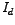) as a function of the voltage across the diode (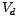) is given by Shockley's equation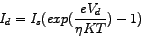'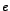' is the electronic charge equaling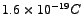,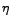is a constant which varies between 1 and 2,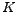is the Boltzmann's constant equaling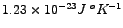,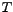is the absolute temperature and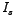is the reverse saturation current.
When the diode is reverse biased (the p and n sides being respectively connected to the -ve and +ve terminals of the battery), the internal barrier field increases (with a corresponding increase in the width of the depletion region), as a result of which the majority charge carriers are further inhibited from crossing the junction and contributing to a current flow. However, the increase in the barrier field helps the minority carriers to cross the junction and contribute to a very small current in the reverse direction. This small current soon attains a constant value with the increase in the reverse bias and is called the reverse saturation current,. It is of the order of nano or pico amperes in Si and micro amperes in Ge. It is important to note that Shockley's equation holds true for the reverse biased diode as well.

###### Numerical solution for the operating point

The operating point of the diode for a fixed set of circuit parameters given by the supply voltage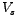and the load resistance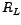, can be obtained by solving simultaneously the diode and load-line equations, viz.,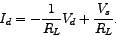The idea, though simple, is difficult to implement, given the nonlinear nature of the diode equation. A way out of the problem is to look for an iterative numerical solution. At firstis eliminated and the above two equations are combined into a single nonlinear equation,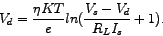A starting guess for, say 0.5 V, is taken and the solution is iteratively launched till the value ofconverges appreciably. This converged value ofis taken as the solution for the diode voltage for the given fixed value ofand. The corresponding diode currentis obtained from either of the two equations considered at the outset.
Another approach, though a bit cumbersome, is to plot the two curves and obtain the point of intersection. This point of intersection would give the same operating point of the diode. The accuracy, though, would be somewhat limited.

###### Effect of temperature on the static curve

One look at Shockley's equation for the diode would tell us that the diode current depends on temperaturethrough its explicit presence inside the exponential. However, we must bear in mind that the reverse saturation currentalso depends on temperature. The dependence (for Si say) can be expressed through the formula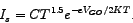C is a constant and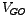is the voltage corresponding to the forbidden energy gap for Si and other symbols have their usual meanings. As a rule of thumb, we may say thatdoubles for every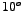rise in temperature. Interestingly, this implicit dependence dominates the explicit dependence mentioned at the outset. The overall effect is that the diode static curve keeps shifting to the left with rise in temperature.

In this context it may be mentioned that Ge diodes have relatively high values of(of the order of micro amperes), rendering them useless over wide temperature fluctuations.

###### Diode resistances

When a diode is subjected to a dc input voltage, a fixed current results and we get a fixed operating point or Q (Qiescent) point on the static diode characteristic curve. The resistance of the diode at this Q point is called the dc or static resistance and is given by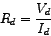whereandare the diode-voltage and diode- current values at this operating point. As one moves up the static point, the dc resistance value goes on decreasing.

On the other hand if the diode is subjected to a small signal alternating (ac) voltage oscillating about a fixed dc value, the current also oscillates about the fixed dc value given by the operating point. The resistance that needs to be considered in this case is the ac or dynamic resistance given by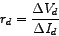where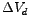and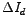are the diode-voltage and diode-current swings about the operating point. We may obtain the ac resistance from the diode static curve (obtained from experiment, say) by drawing a tangent at the operating point and calculatingandtherefrom. Interestingly, by calculating the derivative ofwith respect tofrom Shockley's diode equation, one may arrive at an analytical formula for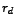, viz.,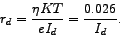The temperature is taken to be the room temperature equaling 300 degree Kelvin. The value of the ac resistance so obtained would be slightly less than that obtained graphically. The difference can be accounted for by considering the resistance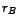of the bulk of the diode and also of the contacts used to connect the diode with the circuit (the dc and ac resistance discussed above were due to the p-n junction only and not due to the bulk of the diode). The bulk resistance can be neglected in comparision to the theoreticaly obtained ac resistance of the diode for low values of diode current.

When the ac voltage swing is large, we need to consider another resistance called the average ac resistance of the diode defined between two extreme operating points of the diode given by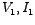and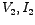as follows: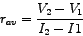It is this ac resistance whose value is used as the slope of the second linear piece in the piecewise linear approximation or model of a diode.